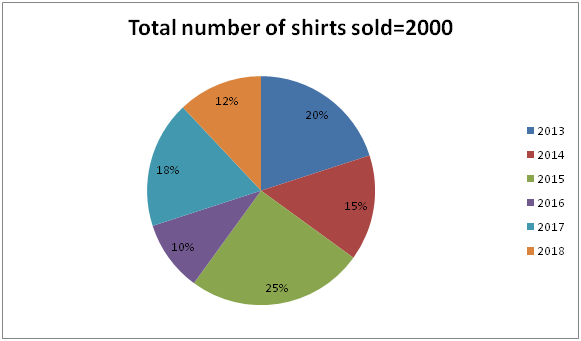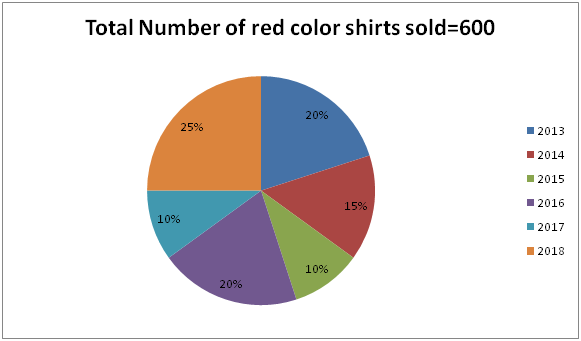# SBI Clerk Mains Quantitative Aptitude (Day-15)

Dear Aspirants, Our IBPS Guide team is providing new series of Quantitative Aptitude Questions for SBI Clerk Mains 2020 so the aspirants can practice it on a daily basis. These questions are framed by our skilled experts after understanding your needs thoroughly. Aspirants can practice these new series questions daily to familiarize with the exact exam pattern and make your preparation effective.

Start Quiz

Ensure Your Ability Before the Exam – Take SBI Clerk 2020 Mains Free Mock Test

Application Sums

1) A boat cover the certain distance in upstream in 6 hours and cover the same distance in downstream in 2 hours. If the speed of the stream is 20 kmph less than that of speed of the boat in still water, then what is the speed of the boat in still water?

a) 20 kmph

b) 40 kmph

c) 60 kmph

d) 30 kmph

e) Cannot be determined

2) If the ratio of the length of the train A and B is 4: 5 and the ratio of the speed of the train A to train B is 3: 4. Train A crosses train B running in opposite direction in 18 seconds and also train B crosses the man standing in a platform in 12 seconds. What is the difference between length of train A and train B?

a) 40 m

b) 80 m

c) 120 m

d) Cannot be determine

e) None of these

3) The bag has 2 apples, 3 bananas and 4 oranges. If three fruits are drawn at random, then what is the probability of drawing exactly 2 oranges?

a) 1/7

b) 3/14

c) 5/14

d) 3/7

e) None of these

4) If the ratio of the height of the cone to the height of the cylinder is 3: 1 and the ratio of the radius of the Cone to cylinder is 1: 2. If the curved surface area of the cone is 550 cm2, what is the total surface area of the cylinder?

a) 724 cm2

b) 684 cm2

c) 268 cm2

d) Cannot be determine

e) None of these

5) Ratio of the length to breadth of the rectangle is 5: 3 and the area of the rhombus is 336 cm2. If the ratio of the diagonals of rhombus is 7: 24 and the side of the rhombus is equal to the length of the rectangle, then what is the area of the rectangle?

a) 255 cm2

b) 335 cm2

c) 225 cm2

d) 375 cm2

e) None of these

Data interpretation

Direction (6 – 10): Study the following information carefully and answer the given questions?

The given pie graph shows the percentage of shirts sold in six different years.The given pie chart shows the percentage of red color shirt sold in six different years.6) If 20% and 30% of the shirts sold during 2014 and 2016 is Green color respectively, then the number of green shirt and red shirt sold during 2014 is approximately what percent of the number of green shirt and red shirt sold during 2016?

a) 81%

b) 83%

c) 85%

d) 87%

e) None of these

7) In 2017, three colors of shirts (Red, Yellow and Blue) are sold and the ratio of the number of shirt sold in Yellow to Blue color in 2017 is 7:8, then what is the difference between the number of Yellow and Blue color shirts sold in 2017?

a) 10

b) 15

c) 20

d) 25

e) None of these

8) What is the ratio of the number of shirts sold in 2013 and 2018 together to the number of red color shirts sold in 2018, 2015 and 2013 together?

a) 60:31

b) 62:33

c) 64:33

d) 65:36

e) None of these

9) The ratio of the yellow to red color shirt sold in 2015 is 5:6 and the ratio of the Yellow to Blue shirt sold in 2015 is 25:28 and what is the difference between the number of yellow and blue shirts sold in 2015?

a) 4

b) 5

c) 6

d) 3

e) None of these

10) What is the approximate average number of shirts (except red) sold in all the years together?

a) 231

b) 233

c) 235

d) 237

e) None of these

Directions (1-5) :

Speed of the boat in still water = x

Speed of the stream = x – 20

Downstream speed = (x + x – 20) = D/(2x – 20)

Upstream speed = x – x + 20 = 20

D/(2x – 20) = 2

D = 4x – 40

D/20 = 6

D = 120 km

120 = 4x – 40

4x = 160

x = 40 kmph

Length of train A = 4x

Length of train B = 5x

Speed of train A = 3y

Speed of train B = 4y

4x + 5x = (3y + 4y) * 5/18 * 18

9x = 35y

5x = 4y * 5/18 * 12

x = 8y/3

3x = 8y

Required probability = 4C2 * 5C1/9C3 = 30/84 = 5/14

Height of the cone = 3x

Height of the cylinder = x

Radius of the cone = y

Radius of the cylinder = 2y

CSA = 22/7 * r * l

Area of the rhombus = 1/2 * (d1 * d2)

336 = 1/2 * (d1 * d2)

672 = 7x * 24x

x = 2 cm

Diagonal1 = 7 * 2 = 14 cm

Diagonal2 = 24 * 2 = 48 cm

Side = √ ((d1/2)2 + (d2/2)2)

= √ (14/2)2 + (48/2)2 = 25 cm

Length of the rectangle = 25 cm

Breadth of the rectangle = 3/5 * 25 = 15 cm

Area of the rectangle = 25 * 15 = 375 cm2

Directions (6-10) :

Green color shirt in 2014 = 300 * 20/100 = 60

Green color shirt in 2016 = 200 * 30/100 = 60

Red color shirt in 2014 = 600 * 15/100= 90

Red color shirt in 2016 = 600 * 20/100 = 120

Required percentage = [(60 + 90)/(120 + 60)] *100 = 83%

Red color shirt in 2017 = 600 * 10/100 = 60

Number of shirts sold in 2017 = 2000 * 18/100 = 360

Number of Yellow and Blue shirts in 2017 = 360 – 60 = 300

The ratio of the number of shirt sold in Yellow to Blue color in 2017 = 7: 8

Difference = 1/15 * 300 = 20

Number of shirts sold in 2013 = 2000 * 20/100 = 400

Number of shirts sold in 2018 = 12/100 * 2000 = 240

Red color shirt sold in 2018 = 600 * 25/100 = 150

Red color shirts sold in 2015 = 600 * 10/100 = 60

Red color shirts sold in 2013 = 600 * 20/100 = 120

Required ratio = (400 + 240): (150 + 60 + 120)

= 640: 330

= 64: 33

Number of red color shirts sold in 2015 = 600 * 10/100 = 60

Number of yellow color sold in 2015 = 5/6 * 60 = 50

Difference = 3/25 * 50 = 6